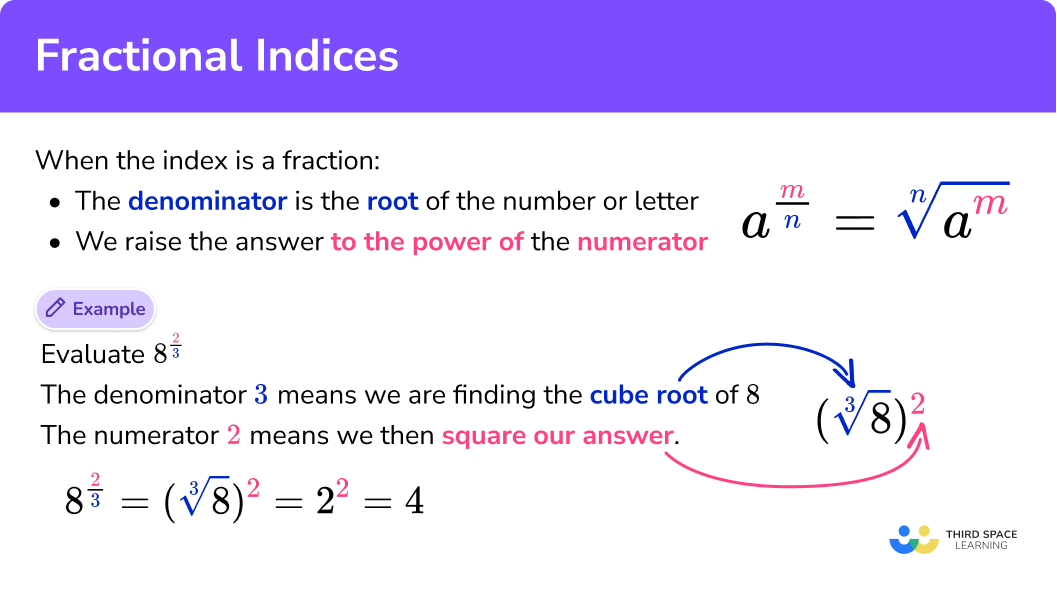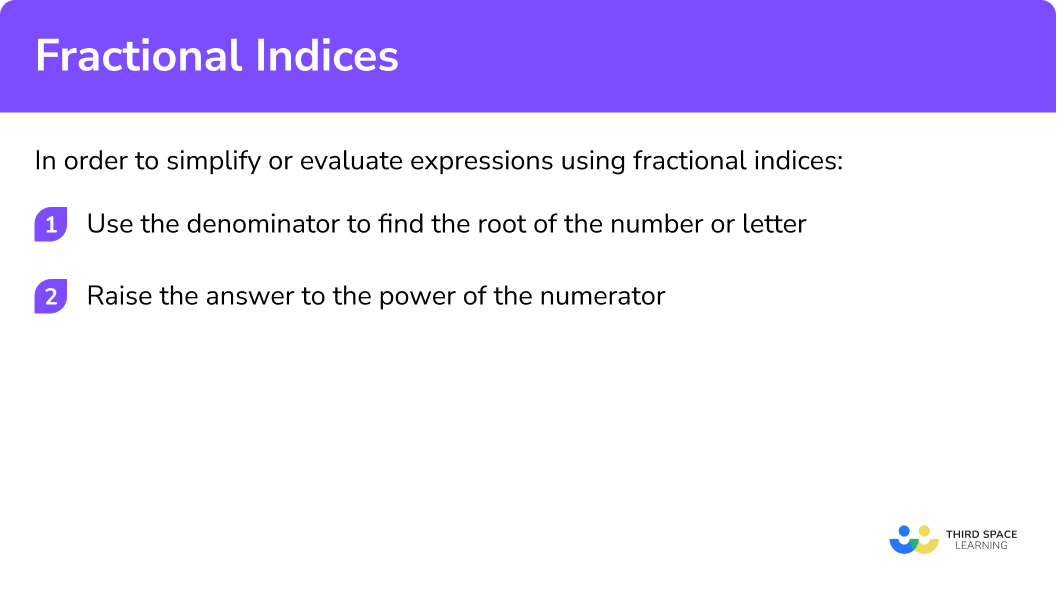GCSE Maths Algebra Law of Indices

Fractional Indices

# Fractional Indices

Here we will learn how to simplify and evaluate with fractional indices for GCSE maths (Edexcel, AQA and OCR).

Look out for the laws of indices worksheets and exam questions at the end.

## What are fractional indices?

Fractional indices are powers of a term that are fractions. Both parts of the fractional power have a meaning.

$x^{\frac{a}{b}}$

The denominator of the fraction (b) is the root of the number or letter.

The numerator of the fraction (a) is the power to raise the answer to.

$(\sqrt[b]{x})^{a}$

### What are fractional indices?### Related laws of indices lessons

Fractional indices is part of the larger topic, laws of indices. It may be useful to explore the main topic before looking into the detailed individual lessons below:

## How to use fractional indices

For example here we have a base number of 8 that has been raised to a fractional power

$8^{\frac{2}{3}}$

As the denominator is 3 we have to find the cube root of 8 .

$\sqrt{8} = 2$

Then, as the numerator is 2 we then square the answer.

$2^{2} = 4$

So,

\begin{aligned} 8^{\frac{2}{3}} &=(\sqrt{8})^{2} \\ &=4 \end{aligned}

• A value raised to the power of ½ means take the square root.
$x^{\frac{1}{2}}=\sqrt{x}$

E.g

$9^{\frac{1}{2}}=\sqrt{9}=\pm{3}$

• A value raised to the power of ⅓ means take the cube root
$x^{\frac{1}{3}}=\sqrt{x}$

E.g

$27^{\frac{1}{3}}=\sqrt{27}=3$

• A value raised to the power of 4 means take the fourth root.
$x^{\frac{1}{4}}=\sqrt{x}$

E.g

$16^{\frac{1}{4}}=\sqrt{16}=\pm{2}$

Etc.

### Explain how to use fractional indices## Fractional indices examples

### Example 1: fractional Indices where the numerator is 1

Simplify

$a^{\frac{1}{4}}$

1. Use the denominator to find the root of the number or letter.

$\sqrt{a}$

2 Raise the answer to the power of the numerator.

In this case the numerator is 1 so the answer stays the same

$\sqrt{a}$

### Example 2: fractional Indices where the numerator is greater than 1

Evaluate

$8^{\frac{2}{3}}$

$\sqrt{8}=2$

$2^{2}=4$

So,

$8^{\frac{2}{3}}=4$

### Example 3: negative fractional Indices

This example uses negative numbers as the indices. It is a good idea to check our Laws of Indices page for more information before attempting this question.

Evaluate

$4^{-\frac{3}{2}}$

First we need to make the index positive by writing the reciprocal.

$4^{-\frac{3}{2}}=\frac{1}{4^{\frac{3}{2}}}$

Then continue to use the steps, focusing on the denominator.

$\sqrt{4}=2$

$2^{3}=8$

So,

$4^{-\frac{3}{2}}=\frac{1}{8}$

### Common misconceptions

• Confusing integer and fractional powers

Raising a term to the power of 2 means we square it

E.g

$a^{2}=a \times a$

Raising a term to the power of ½ means we find the square root of it

E.g

$a^{\frac{1}{2}}=\pm \sqrt{a}$

Raising a term to the power of 3 means we cube it

E.g

$a^{3}=a \times a \times a$

Raising a term to the power of ⅓ means we find the cube root of it

E.g

$a^{\frac{1}{3}}=\sqrt{a}$

• Indices, powers or exponents

Indices can also be called powers or exponents.

### Practice fractional indices questions

64^{\frac{1}{2}}

\pm \; 128\pm \; 8\pm \; 32\pm \; 4The index number tells us to find the square root, so

64^{\frac{1}{2}}=\sqrt{64}=8

27^{\frac{2}{3}}

189354Looking at the index number, the denominator tells us to cube root, and the numerator tells us to square, therefore

\begin{aligned} &27^{\frac{2}{3}}\\ &=(\sqrt{27})^{2}\\ &=3^{2}\\ &=9 \end{aligned}

64^{-\frac{3}{2}}

\frac{1}{8}\frac{1}{64}\frac{1}{512}\frac{1}{16}Looking at the index number, the denominator tells us to square root, the numerator tells us to cube, and as the index is negative we find the reciprocal, therefore

\begin{aligned} &64^{-\frac{3}{2}}\\ &=(\sqrt{64})^{-3}\\ &=\frac{1}{8^{3}}\\ &=\frac{1}{512} \end{aligned}

4. Evaluate

81^{\frac{3}{4}}

9273243Looking at the index number, the denominator tells us to take the fourth root, and the numerator tells us to cube, therefore

\begin{aligned} &81^{\frac{3}{4}}\\ &=(\sqrt{81})^{3}\\ &=3^{3}\\ &=27 \end{aligned}

### Fractional indices GCSE questions

1. Evaluate

9^{\frac{1}{2}}

(1 mark)

\pm 3

(1)

2. Evaluate

(27)^{\frac{2}{3}}

(2 marks)

(\sqrt{27})^{2} \\

(1)

9

(1)

3. Evaluate

\left(\frac{16}{81}\right)^{-\frac{3}{4}}

(3 marks)

\left(\sqrt{\frac{81}{16}}\right)^{3} \\

(1)

\left(\frac{3}{2}\right)^{3} \\

(1)

\frac{27}{8}=3.375

(1)

## Learning checklist

You have now learned how to:

• Simplify expressions involving the laws of indices
• Calculate with roots, and with integer and fractional indices

## Still stuck?

Prepare your KS4 students for maths GCSEs success with Third Space Learning. Weekly online one to one GCSE maths revision lessons delivered by expert maths tutors.

Find out more about our GCSE maths tuition programme.